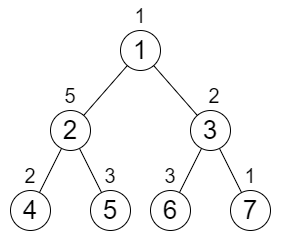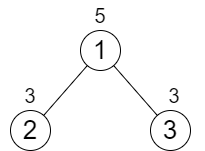2673. Make Costs of Paths Equal in a Binary Tree
Medium
374
3

You are given an integer `n` representing the number of nodes in a perfect binary tree consisting of nodes numbered from `1` to `n`. The root of the tree is node `1` and each node `i` in the tree has two children where the left child is the node `2 * i` and the right child is `2 * i + 1`.

Each node in the tree also has a cost represented by a given 0-indexed integer array `cost` of size `n` where `cost[i]` is the cost of node `i + 1`. You are allowed to increment the cost of any node by `1` any number of times.

Return the minimum number of increments you need to make the cost of paths from the root to each leaf node equal.

Note:

• A perfect binary tree is a tree where each node, except the leaf nodes, has exactly 2 children.
• The cost of a path is the sum of costs of nodes in the path.

Example 1:```Input: n = 7, cost = [1,5,2,2,3,3,1]
Output: 6
Explanation: We can do the following increments:
- Increase the cost of node 4 one time.
- Increase the cost of node 3 three times.
- Increase the cost of node 7 two times.
Each path from the root to a leaf will have a total cost of 9.
The total increments we did is 1 + 3 + 2 = 6.
It can be shown that this is the minimum answer we can achieve.
```

Example 2:```Input: n = 3, cost = [5,3,3]
Output: 0
Explanation: The two paths already have equal total costs, so no increments are needed.
```

Constraints:

• `3 <= n <= 105`
• `n + 1` is a power of `2`
• `cost.length == n`
• `1 <= cost[i] <= 104`
Accepted
9.3K
Submissions
15.7K
Acceptance Rate
59.1%

Seen this question in a real interview before?
1/4
Yes
No

Discussion (0)

Related Topics